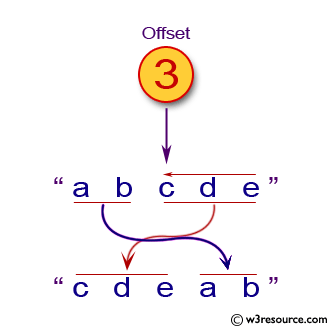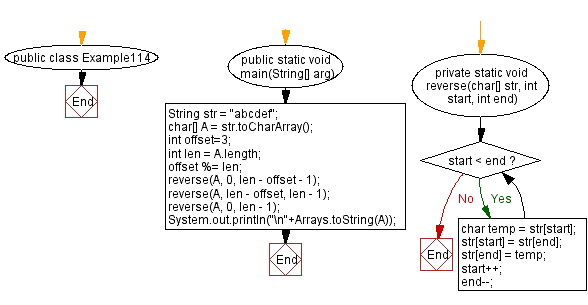﻿ Java exercises: Given a string and an offset, rotate string by offset - w3resource# Java Exercises: Given a string and an offset, rotate string by offset

## Java Basic: Exercise-114 with Solution

Write a Java program to given a string and an offset, rotate string by offset (rotate from left to right).

Pictorial Presentation:Sample Solution:

Java Code:

``````import java.util.*;
import java.util.*;
public class Example114 {
public static void main(String[] arg)
{
String str = "abcdef";
char[] A = str.toCharArray();
int offset=3;
int len = A.length;
offset %= len;
reverse(A, 0, len - offset - 1);
reverse(A, len - offset, len - 1);
reverse(A, 0, len - 1);
System.out.println("\n"+Arrays.toString(A));
}

private static void reverse(char[] str, int start, int end) {
while (start < end) {
char temp = str[start];
str[start] = str[end];
str[end] = temp;
start++;
end--;
}
}
}
```
```

Sample Output:

```[d, e, f, a, b, c]
```

Flowchart:Java Code Editor:

What is the difficulty level of this exercise?

﻿

## Java: Tips of the Day

initial:

Returns all the elements of an array except the last one. Use Arrays.copyOfRange() to return all except the last one

```public static <T> T[] initial(T[] elements) {
return Arrays.copyOfRange(elements, 0, elements.length - 1);
}
```

Ref: https://bit.ly/3zEKw9u